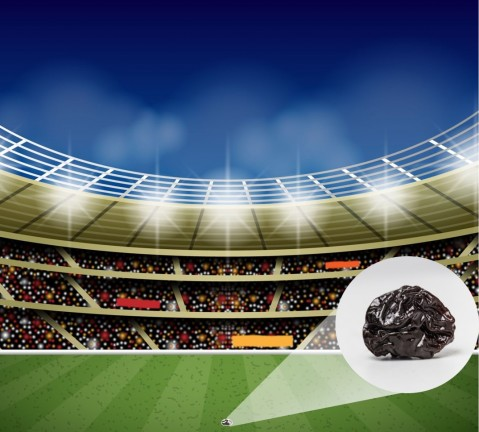ONLINE  ZOOM tution : Mob: +44 (0)7557331782 :  david@mychem.co.uk

# 1.26 - 1.28 Moles and mountains

#### 1.26 "Counting" by measuring mass

Large numbers of coins can be counted by measuring their mass

​Sometimes chemists need to "count" out a specific number of atoms, ions or molecules of a substance. Atoms are very very small - too small to see. It is therefore impossible to measure them out by counting individually.  Instead we "count" them out by measuring their mass - much like the way in which coins are counted out in banks by using weighing machines.

We know the Relative atomic masses ( or Ar) of atoms.  This is usually  shown next to the symbol of the element  on the periodic table.

Assumed background knowledge:### 1.14 - 1.17 Atomic structure

​If a nucleus was the size of a raisin, the rest of the atom would be the size of a sports stadium

Relative atomic masses are usually shown next to the element's symbol on the  periodic table.

#### Students should:

1.25 write word equations and balanced chemical equations (including state symbols):

• for reactions studied in this specification
• for unfamiliar reactions where suitable information is provided

1.26 calculate relative formula masses (including relative molecular masses) (Mr) from relative atomic masses (Ar)

#### 1.26 Calculating formula mass

The mass of one mole of a compound can be calculated using the relative atomic masses of the component elements multiplied by the number of atoms shown in the formula.

#### 1.27 What is a mole?

A mole of rice grains would form a huge mountain of rice with enough rice to feed the world's current population for the next 6088978 years!

#### Students should:

1.27 know that the mole (mol) is the unit for the amount of a substance

1.28 understand how to carry out calculations involving amount of substance, relative atomic mass (Ar) and relative formula mass (Mr)

A mole (mol) is the quantity of anything that has the same number of particles as there are atoms found in 12.0 grams of carbon-12.

This number of particles is a very large number : 6 x 1023

One mole of atoms of an element is the amount of that element equal to the relative atomic mass in g

In other words - one mole of oxygen atoms has a mass of 16g, one mole of Lithium atoms has a mass of 6.9g etc.

Thus there are the same number of atoms in 16g of oxygen as there are atoms in 6.9g  Lithium and of course 12g  of Carbon.

#### 1.28 Calculating moles

Calculating the number of moles knowing the mass and the Ar ( mass of one mole)

The following masses of elements all contain one mole of atoms:

• 12.0 g Carbon,
• 32.1 g Sulphur,
• 14 g Nitrogen,
• 24.3 g Magnesium.

So if you have 24 g carbon you have 24/12 = 2 moles carbon atoms.

Likewise if you have 1.4 g nitrogen you have 1.4/14 = 0.1 moles nitrogen atoms.

#### Rearranging things

The mass of one mole ("MOOM")is the relative atomic number for an element. If the substance is a compound you will need to work out its relative molecular mass or relative formula mass.

The relationship between the mass in g ("MIG") of a sample, the number of moles ("NOM") of substance an the mass of  one mole ("MOOM") of a substance  can be shown as a triangle:

Cover up the quantity you want to find to give the appropriate formula for calculating that quantity.

#### Activity 1. Moles from mass

Watch the short video to see how the number of moles can be calculated from the mass of the sample and the mass of one mole (Ar) . Use the same method to calculate the number of moles in the five samples given on the questions tab below. Use the answers tab to check your calculations:

How many moles of atoms are there in :
1. 355g of chlorine
2. 0.69g of lithium
3. 8.0g of oxygen
4. 35g of copper
5. one tonne of lead

There are:

1. 10
2. 0.1
3. 0.5
4. 0.55
5. 4826.25

moles of atoms

#### Activity 2. Mass from moles

Watch the short video to see how the mass of a sample can be calculated from the number of moles and the mass of one mole (Ar) . Use the same method to calculate the mass in the ten samples given on the questions tab below. Use the answers tab to check your calculations:

What is the mass of:

1. 2.5 moles of oxygen atoms?
2. 0.15 moles boron atoms
3. 5 moles of aluminium atoms
4. 0.4 moles of sulfur atoms
5. 50 moles of oxygen atoms
6. 1 mole of water molecules
7. 0.5 moles of ethanol
8. 4 moles of glucose
9. 2 moles of sodium chloride
10. 2 moles of carbon dioxide molecules

Enter your text here ...

Enter your text here ...

Enter your text here ...

Enter your text here ...

Enter your text here ...

The masses are:

1. ​2.5 x 16 = 40g
2. 0.15 x 10.8 = 1.62g
3. 5 x 27 = 135g
4. 0.4 x 32.1 = 12.84g
5. 50 x 16 = 800g
6. 1 x 18 = 18g
7. 0.5 x 46 =  23g
8. 4 x 180 = 720g
9. 2 x 58.5 = 117g
10. 2 x 44 = 88g

#### Activity 3. Matching moles and masses

When you have successfully completed the calculations in activities 1 and 2 you should be able to test your skill using the quizlet below:

Comment for this post has been locked by admin.

#### Comments

By accepting you will be accessing a service provided by a third-party external to https://mychem.co.uk/